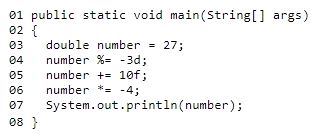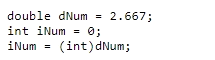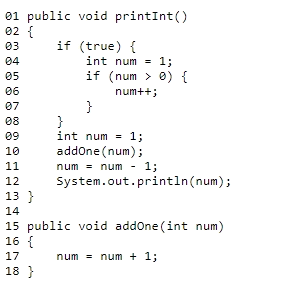# Microsoft 98-388 Introduction to Programming Using Java Exam Practice Test

Page: 1 / 14
Total 44 questions

### Question 1

You have the following code segment. Line numbers are included for reference only.What is the output of line 07?

### Question 2

This question requires that you evaluate the underlined text to determine if it is correct.

You should use an int data type to store the numeric value 3,000,000,000 (3 billion) so that the least amount of memory is used.

Review the underlined text. If it makes the statement correct select "No change is needed." If the statement is incorrect, select the answer choice that makes the statement correct.

### Question 3

You need to evaluate the following code segment:What happens when the code segment is run?

### Question 4

You work as a Java programmer.

You need to convert a numeric string to a primitive double value.

Which code segment should you use?

### Question 5

You need to analyze the following code segment. Line numbers are included for reference only.What is the output of line 12 when you run printint()?